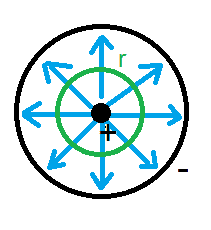# Electric field with electric potential

## Homework Statement

A metallic hollow cylinder has a diameter of ##4.2 cm##. Along his axis there is a wire having a diameter of ##2.68 \mu m##(considering it as a hollow cylinder). Between the cylinder and the wire there is a voltage of ##855 V##.
What is the electric field on the wire surface and the cylinder surface?

## Homework Equations

Electric potential:
##\int_{A}^{B} \vec E_0 \cdot d\vec l = V_0(A) - V_0(B)##
For a generic point ##P##
##V_0(x, y, z) = \int_A^P \vec E_0 \cdot d\vec l + V_0(A)##

## The Attempt at a Solution

First I got the radius from the diameters, so:
##D_1 = 2.68\mu m = 2.68 * 10^-6 m##
##D_2 = 4.2 cm = 4.2 * 10^-2 m##
##R_1 = 1.34 * 10^-6 m##
##R_2 = 2.1 * 10^-2 m##

At this point I know that ##V_0(R_1) - V_0(R_2) = 855 V##, so I have to use the first equation to find out ##E_0##. The problem is that I don't understand what ##E_0## I am calculating with this equation:
$$\int_{R_1}^{R_2} \vec E_0 \cdot d\vec l = V_0(R_1) - V_0(R_2)$$
Is it the wire surface? Or am I calculating the electric field inside the cylinder but outside the wire?

Simon Bridge
Homework Helper
You could try the differential form: ##\vec E=-\vec \nabla V## and select a suitable coordinate system and boundary conditions.

Boundary conditions? What do you mean by that?

ehild
Homework Helper

## Homework Statement

A metallic hollow cylinder has a diameter of ##4.2 cm##. Along his axis there is a wire having a diameter of ##2.68 \mu m##(considering it as a hollow cylinder). Between the cylinder and the wire there is a voltage of ##855 V##.
What is the electric field on the wire surface and the cylinder surface?

## Homework Equations

Electric potential:
##\int_{A}^{B} \vec E_0 \cdot d\vec l = V_0(A) - V_0(B)##
For a generic point ##P##
##V_0(x, y, z) = \int_A^P \vec E_0 \cdot d\vec l + V_0(A)##

At this point I know that ##V_0(R_1) - V_0(R_2) = 855 V##, so I have to use the first equation to find out ##E_0##. The problem is that I don't understand what ##E_0## I am calculating with this equation:
Where is that equation from? You should use only such formulas which are explained.
You certainly know that the line integral between two points does not depend on the path taken and ##\int_{A}^{B} \vec E \cdot d\vec l = V(A) - V(B)## where the integral goes along the radius now, from the wire surface to the surface of the cylinder. You have to know how the electric field depends on the radius. For that, use Gauss' Law.

Simon Bridge
Homework Helper
Boundary conditions? What do you mean by that?
Boundary conditions are the conditions on the boundary of the problem. Your problem has two boundaries - which is the cylinder surfaces.
The relevant condition on those boundaries is the potentials there.
Do you not know how to solve differential equations?

Do you know how to use cylindrical-polar coordinates?
Do you know Gauss' Law?

Differential equations are those where you derive for each unknown and take the others as constant. (x, y, z in this case, since we have three coordinates)
I never used the cylindrical and polar coordinates but I read about them in the book.
Yes, I know the Gauss' Theorem.

You have to know how the electric field depends on the radius. For that, use Gauss' Law.
But how? I don't know the charge distribution.

ehild
Homework Helper
Differential equations are those where you derive for each unknown and take the others as constant. (x, y, z in this case, since we have three coordinates)
I never used the cylindrical and polar coordinates but I read about them in the book.
Yes, I know the Gauss' Theorem.

But how? I don't know the charge distribution.
But you know the symmetry of the problem. You can assume that the charge distribution is even, the charge is λ on unit length of the wire.
And you know the potential difference between the metallic surfaces. The metallic surfaces are equipotentials, and the electric field is perpendicular to them, and is the same in every direction. The blue lines show the electric field lines, and the grin circle is the cross section of a Gaussian cylinder. Apply Gauss Law to get the electric field intensity at distance r from the axis.